Search

Solving Inequalities: An Overview (page 3 of 3)

Sections: Linear inequalities, Quadratic inequalities, Other inequalities

General Polynomial Inequalities

• Solve x5 + 3x4  23x3  51x2 + 94x + 120 > 0

First, I factor to find the zeroes:

x5 + 3x4  23x3  51x2 + 94x + 120

= (x + 5)(x + 3)(x + 1)(x  2)(x  4) = 0

...so x = 5, 3, 1, 2, and 4 are the zeroes of this polynomial. (Review how to solve polynomials, if you're not sure how to get this solution.)

To solve by the Test-Point Method, I would pick a sample point in each interval, the intervals being (negative infinity, 5), (5, 3), (3, 1), (1, 2), (2, 4), and (4, positive infinity). As you can see, if your polynomial or rational function has many factors, the Test-Point Method can become quite time-consuming.

To solve by the Factor Method, I would solve each factor for its positivity: x + 5 > 0 for x > 5; x + 3 > 0 for x > 3; x + 1 > 0 for x > 1; x  2 > 0 for x > 2; and x  4 > 0 for x > 4. Then I draw the grid: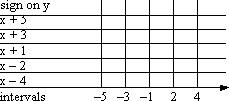...and fill it in: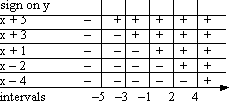...and solve: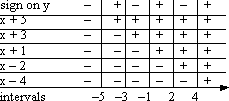Then the solution (remembering to include the endpoints, because this is an "or equal to" inequality) is the set of x-values in the intervals [5, 3], [1, 2], and [4, positive infinity]

As you can see, if your polynomial or rational function has many factors, the Factor Method can be much faster.

Rational Inequalities

• Solve x/(x  3) < 2

First off, I have to remember that I can't begin solving until I have the inequality in "= 0" format.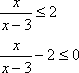Now I need to convert to a common denominator: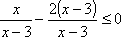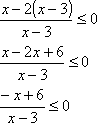The two factors are x + 6 and x  3. Note that x cannot equal 3, or else I would be dividing by zero, which is not allowed. The first factor, x + 6, equals zero when x = 6. The other factor, x  3, equals zero when x = 3. Now, x cannot actually equal 3, so this endpoint will not be included in any solution interval (even though this is an "or equal to" inequality), but I need the value in order to figure out what my intervals are. In this case, my intervals are (negative infinity, 3), (3, 6], and [6, positive infinity). Note the use of brackets to indicate that 6 can be included in the solution, but that 3 cannot.

Using the Test-Point Method, I would pick a point in each interval and test for the sign on the result. I could use, say, x = 0, x = 4, and x = 7.

Using the Factor Method, I solve each factor: x + 6 > 0 for x > 6, or x < 6; x  3 > 0 for x > 3. Then I do the grid: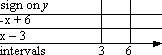...fill in the signs on the factors: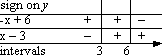...and solve for the sign on the rational function: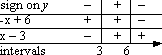So the solution is all x's in the intervals (negative infinity, 3) and [6, positive infinity).

There is another way to solve inequalities. You still have to find the zeroes (x-intercepts) first, but then you graph the function, and just look: wherever the graph is above the x-axis, the function is positive; wherever it is below the axis, the function is negative. For instance, for the first quadratic exercise, y = x2  3x + 2 > 0, we found the zeroes at x = 1 and x = 2. Now look at the graph: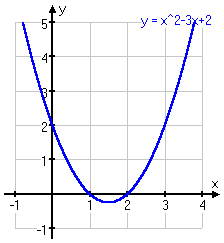On the graph, the solution is obvious: you would take the two intervals (but not the interval endpoints) where the line is above the x-axis.

Or that huge polynomial we did at the top of this page: x5 + 3x4  23x3  51x2 + 94x + 120 > 0. We found the zeroes of the polynomial, being x = 5, x = 3, x = 1, x = 2, and x = 4. Now look at the graph: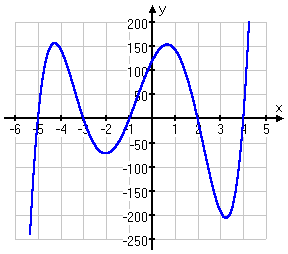On the picture, the solution is obvious: take the three intervals (together with the interval endpoints) where the line is above the x-axis.

As you can probably guess, a graphing calculator can save you a lot of time on these inequalities  if you understand what you're doing. You should still show your work and reasoning, but don't be shy about using the pictures to confirm the algebra.

<< Previous  Top  |  1 | 2 | 3  |  Return to Index

 Cite this article as: Stapel, Elizabeth. "Solving Inequalities: An Overview." Purplemath. Available from     https://www.purplemath.com/modules/ineqsolv3.htm. Accessed [Date] [Month] 2016

Reviews of
Internet Sites:
Free Help
Practice
Et Cetera

Study Skills Survey

Tutoring from Purplemath
Find a local math tutor